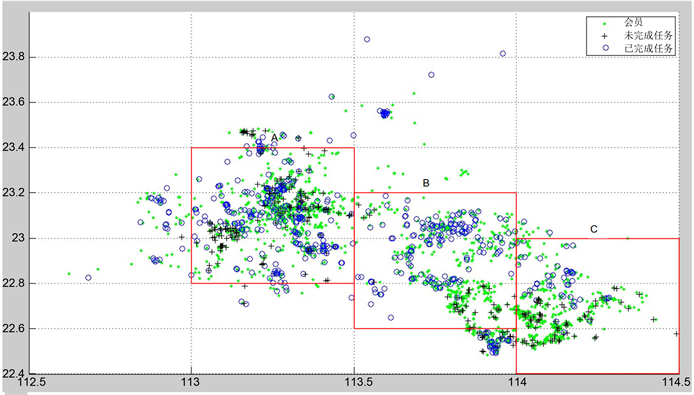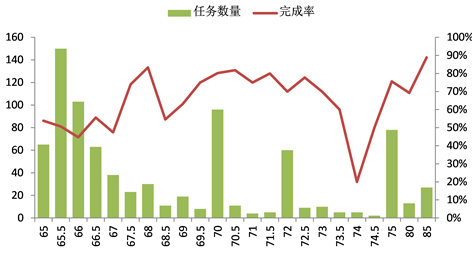# 基于区域定价模型的劳务众包任务定价方案Labor Crowd Sourcing Pricing Based on Regional Pricing Model

DOI: 10.12677/ECL.2018.72003, PDF, HTML, XML, 下载: 663  浏览: 1,732  科研立项经费支持

Abstract: Internet service crowd sourcing platform has promoted business task pricing. Traditional pricing schemes are easily affected by geographical distribution, membership number and reputation value, which results in low task completion rate in some areas. Based on the regional pricing model and task packing scheme, the task is redefined. Logistic regression is used to determine the completion rate equation, and the new task pattern is formed through the systematic cluster. Taking the parameters such as member concentration as independent variables, regression analysis is applied to get different regional task pricing equations. The completion rate is simulated to form a new pricing model. The new pricing is more reasonable. The results of model evaluation show that the model can effectively improve the task completion rate, reduce cost, improve the transaction efficiency, and increase the revenue for the platform.

1. 研究背景

2. 定价模型

2.1. 价格影响因素Figure 1. The distribution of tasks and members

2.1.1. 任务密集程度

2.1.2. 会员密集程度

${d}_{AB}=\frac{\text{π}R}{180}\sqrt{{\left({\theta }_{A}-{\theta }_{B}\right)}^{2}+{\left({\phi }_{A}-{\phi }_{B}\right)}^{2}}$ (1)

1) 任务分布密集程度与定价水平负相关；

2) 会员分布密集程度与定价水平负相关；

3) 任务密集区周边，会员分布较稀疏地区任务定价较高。Figure 2. The tasks and mean prices of different numbers of members within 1 kmTable 1. Basic information of tasks and members in A, B, C

2.1.3. 任务完成率

2.2. 定价模型

2.2.1. 区域定价方程Figure 3. The completion quantities and rates of tasks at each price

1) 人均任务量： $\rho =\frac{{T}_{i}}{{M}_{i}}$ ；2) 信誉程度： ${C}_{i}=\frac{\underset{i}{\sum }{c}_{ij}}{{M}_{i}}$ ；3) 限额水平： ${L}_{ij}=\frac{\underset{j}{\sum }{l}_{ij}}{{M}_{i}}$

$P=\alpha {M}_{i}+\beta$ (2)

${F}_{i}=\varphi {M}_{i}+\phi {l}_{ij}+\delta {r}_{i}$ (3)

${p}_{i}=\frac{1}{1+{\text{e}}^{-{f}_{i}}}$ (4)

2.2.2. 任务打包

1) 层次聚类

2) 打包后的任务定价

3. 模型评价

4. 总结Table 2. The comparison before and after optimized prices

  Jeff, H. (2006) The Rise of Crowd Sourcing. Wired, 14, 1-4.  顾姝姝, 陈曦. 众包平台研究综述与众包平台绩效影响机制构建[J]. 科技进步与对策, 2017, 34(22): 153-160.  冯剑红, 李国良, 冯建华. 众包技术研究综述[J]. 计算机学报, 2015, 38(9): 1713-1726.  陈瑞泽, 周正, 朱力. 众包平台的任务定价规律模型[J]. 经贸实践, 2017(21): 308.  浦东平, 樊重俊, 袁光辉, 杨云鹏. 精英蜂群算法及考虑利益相关者的众包定价模型[J]. 计算机应用研究, 2019(5): 1-9.  Mason, W. and Watts, D.J. (2010) Financial Incentives and the “Performance of Crowds”. ACM SIGKDD Explorations Newsletter, 11, 100-108.https://doi.org/10.1145/1809400.1809422  全国大学生数学建模竞赛. 2017年高教杯全国大学生数学建模竞赛B题[EB/OL]. http://mcm.blyun.com/  Digout, J., Azouri, M., Decaudin, J.-M. and Rochard, S. (2013) Crowd Sourcing. Outsourcing to Obtain a Creativity Group. Arab Economic and Business Journal, 8, 1-2. https://doi.org/10.1016/j.aebj.2013.11.001  夏恩君, 赵轩维, 李森. 国外众包研究现状和趋势[J]. 技术经济, 2015, 34(1): 28-36.  黄怡淳. 科研众包创新平台的运作机制研究及其可持续发展的经验总结[J]. 科技管理研究, 2017, 37(23): 125-129.  Vukovic, M. and Das, R. (2013) Decision Making in Enterprise Crowdsourcing Services. Service-Oriented Computing. ICSOC 2013. Lecture Notes in Computer Science, Vol. 8274. Springer, Berlin, Heidelberg, 624-638.  Ali-Hassan, H. and Allam, H. (2016) Comparing Crowd Sourcing Initiatives: Toward a Typology Development. Canadian Journal of Ad-ministrative Sciences/Revue Canadienne des Sciences de l’Administration, 33, 4.  Przybylska, N. (2014) Crowdsourcing as a Method of Obtaining Information. Foundations of Management, 5, 1.  孟韬, 张媛, 董大海. 基于威客模式的众包参与行为影响因素研究[J]. 中国软科学, 2014(12): 112-123.  张利斌, 钟复平, 涂慧. 众包问题研究综述[J]. 科技进步与对策, 2012, 29(6): 154-160.